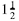top of page

# How to Use Negative Fractions in Equations

A negative fraction is a fraction with a negative sign in front of it. Let's show you how to apply negative fractions to equations.

What Is a Negative Fraction?

Subtracting fractions and applying a negative fraction to an equation work the same way:Negative fractions example formula

When you subtract a number, you are taking its value away from another number. Sometimes, that can make the final number less than 0. This is demonstrated in the number line below:Adding two numbers is combining their values. In the number line above, you are adding the value of positive 1 and negative, which is the same thing as subtracting 1.

When you subtract a negative fraction, the minus sign cancels with the subtraction sign. This is why the original fraction turns into a positive number:Subtractive negative fractions

A similar thing happens when you multiply fractions that are both negative numbers:Multiplying fractions

When you look at the front of the fractions within these parentheses, they both have negative signs. When you multiply two negative fractions (or any two negative numbers), the result will be a positive number.

Examples of Negative Fractions

Let's solve these negative fraction math problems that involve improper fractions and the lowest common denominator:Improper negative fractions

The above fractions are improper fractions because each has a top number (the numerator) that’s bigger than the bottom number (the denominator). They also have unlike denominators so we need to find the lowest common denominator, which is the lowest term that both 7 and 14 are divisible by:Lowest common denominator

Since 7 and 14 are both divisible by 28, this will be the lowest common denominator. Now, let’s multiply the numerators and denominators to get the denominators of 28.Multiply the numerators and denominators

Now that these are equivalent fractions (they have the same denominator) we can proceed with this negative fraction:Equivalent fractions

We can further simplify this negative fraction by dividing both values by 14, since 14 goes evenly into both 14 and 28:Simplify the fractions

When Will You Use Negative Fractions?

Subtracting a fraction is the same thing as adding a negative fraction. And multiplying two negative fractions always results in a positive answer. Knowing how to use negative fractions helps you better understand how to combine fractional values and may be useful in your everyday activities like cooking.